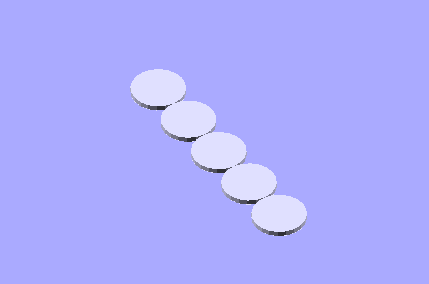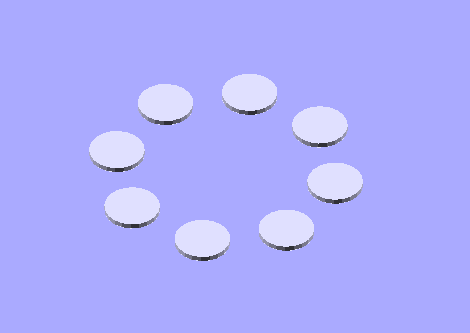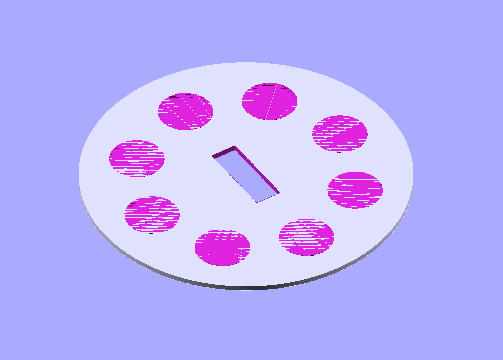One of the strengths of OpenSCAD is it’s ability to parametrically handle parts. Although this is pretty straight forward when it comes to modifying parts lengths and linear values using variables, it may not be as clear where to start with circular parts. This article will examine this, and look deeper into the OpenSCAD language as a continuation of our other article, “Using OpenSCAD for 3D Printing”. If you have not read that article yet, and haven’t used OpenSCAD before, you may want to look over that before proceeding.

There are a few different ways of working with curves and circles in OpenSCAD. We’re going take a more algorithmic approach to working with them. This is in part to illustrate some aspects of the language's syntax, and in part to show how to work with a model when one of the built-in modules does not seem to do the job you need it to do.

First, let’s examine loops, and how to use them when dealing with circular parts.

Loops

Loops allow us to execute a block of code over and over again. OpenSCAD has one kind of loop, called a “for loop”. For loops take a given set of values and iterate through each value once. While it is looping, you can access the value it is currently is on.

Let’s take a look at a simple example. Start OpenSCAD, and enter the following in the text area on the left:

```for (i=[1:5])  {
translate([i,0,0]) cylinder(r=.5,h=.1,\$fn=20);
}```

Press F5 to compile the code. You should see a string of 5 small cylinders in a row.With each iteration “i” is increasing by one. Since “i” is being used to determine the placement on the X-axis, this is causing a cylinder to be placed 1 mm further along on that axis. After it’s done with the last iteration (“5”) it stops executing that block of code, which means it no longer places cylinders contained in that block.

Now let’s try applying this to a circle. This is going to be very similar, but we’re going to use some of the built-in trig functions to place them in a circular path. Open a new file, and enter this code:

```pathRadius=2;
num=8;
for (i=[1:num])  {
}```

Press F5 to compile this, and you should see 8 cylinders like before, this time in a circle. You can try changing the number of circles and the radius by changing the variables “pathRadius” and “num”.How is this different from the linear example from before? First, the for loop is set to go from one to “num”, giving us greater control over how many circles we make. Second, the translate statement is using sine and cosine to compute the location. Finally, we’re using “num” in the sine and cosine functions to determine how many degrees our current cylinder iteration should be placed at by dividing 360 degrees by the number of cylinders:

`translate([pathRadius*cos(i*(360/num)),pathRadius*sin(i*(360/num)),0])`

The variable “pathRadius” is just providing the radius from the center of the group to the center of the individual circles.

Now let’s make this a little more useful, and wrap this in a difference block, where the individual cylinders are cut out of a larger cylinder. We’ll also cut out a slot in the center of the whole thing. Start a new file, and enter this code:

```pathRadius=2;
num=8;
difference(){
cylinder(r=3, h=.1, \$fn=204);
for (i=[1:num])  {
}
cube(size=[1.5,.5,1], center=true);
}```

Press F5 to render this again. Now that’s beginning to look like a part we could possibly use.Conditions

Let’s assume this is a spacer between two other parts. Posts along the outside are going to hold this in place, and later on another part will be added to fit through the center slot. First, to make this more reasonable, I’m going to scale all the sizes up by 10. This may still be a little tricky for your printer, depending on how well calibrated it is, so if you want to print this you may consider resizing it in your print software before your print it.

Also, there’s another problem, mainly the orientation of the slot. Since all of the circles are the same size, the part could be oriented in any direction, changing the relative angle of the slot to other parts. If we pick one hole and make it larger (with a corresponding larger post), then it would solve this problem.

Lets look at some code that solves this problem, and corrects the scaling issue. Start a new file, and enter this code:

```pathRadius=20;
num=8;
difference(){
cylinder(r=30, h=1, \$fn=204);
for (i=[1:num])  {
if (i==1) {
} else {
}
}
cube(size=[15,5,10], center=true);
} ```

Press F5 to compile.The scaling change is pretty straight forward. What’s interesting is the if...else code blocks we added. This checks to see if we’re on the first circle. If it is (i.e. if our variable “i” equals 1), it uses a slightly larger radius. Otherwise (as shown by the “else” block) it will just create the same size hole as we’ve used before. But what if we want multiple holes resized? You can just follow with other nested if statements like:

```if () {
...
}else{
if() {
...
}else{
...
}
}```

Another technique is to check against the modulus operator ("%"). Modulus is division, but it gives us the remainder instead of the actual divided number. So, for example this will make every other hole larger:

```pathRadius=20;
num=8;
difference(){
cylinder(r=30, h=1, \$fn=204);
for (i=[1:num])  {
if ((i%2)==1) {
}else {

}
}
cube(size=[15,5,10], center=true);
}```Modules

By this point, our code is beginning to look a little convoluted. An easy way to organize it is to split it up into separate parts called models. This will also allow us to easily reuse the the code multiple times to create similar objects and modifications. As an example, enter and compile this code as a new document:

```pathRadius=20;
numHoles=8;

for (i=[1:num])  {
if ((i%2)==1) {
}else {

}
}
}

difference(){
cylinder(r=40, h=1, \$fn=204);
cube(size=[15,5,10], center=true);
}```We're defining the module "holes" first, before our main code defining the rest of the part. The definition includes the variables inside the module in parenthesis, including a default value. We can change this later on by setting the variable when we use (call) the module.

The actual call is just the identifier ("holes") with reassigned variables in parenthesis:

`holes(pRad=(pathRadius/2),num=(numHoles/2),circRad=2);`

Now we can reuse the code repeatedly. For this part, we're adding a second set of holes inside the first.

Modules, loops, and conditions give us new powerful ways of working with our part code. For more information check out the other tutorials available on Prinstspace3D, openscad.org, and the OpenSCAD manual available on wikibooks. Have fun making your next printed part!